# Important Question for Class 12 Maths Chapter 4 - Determinants

Important questions for Class 12 Maths Chapter 4 determinants are given here. These questions cover several short answer type questions, long answer type questions that are crucial for CBSE class 12 exams. Also, additional important questions are also provided here. Students can practice these problems for the board examinations. Get all the important Questions for Class 12 Maths Chapters at BYJU’S.

As per the CBSE syllabus, the important questions for class 12 chapter 4 are given. In this chapter, the following concepts are covered. It covers the basic concept of determinants and its properties, adjoint and inverse of matrices, cofactors and minors, and the applications of determinants. It covers all the type of questions such as 1 mark, 2 marks, 5 marks questions from these topics. Let us discuss the introduction of determinants here.

What are Determinants?

A determinant is defined as a numerical value of the square matrix. It is represented by det A or |A|. For every square matrix, A =[aij] of order n, we can associate a number with either real number or complex number, called the determinant of the square matrix.

Singular and Non-singular Matrix:

A matrix is said to be a square matrix if the value of the determinants corresponds to the square matrix is zero. Otherwise, the matrix is called the non-singular matrix.

It means that,

|A| = 0, then the matrix is a singular matrix

|A|≠ 0, then the matrix is called a non-singular matrix.

Also, Check:

## Class 12 Chapter 4 Determinants Important Questions with Solutions

Here, the important questions for class 12 chapter 4 determinants are given here with solutions. The solutions are given in a step-by-step procedure so that students can understand in an easy and a better way. Go through the solved problems and score good marks in class 12 final examination.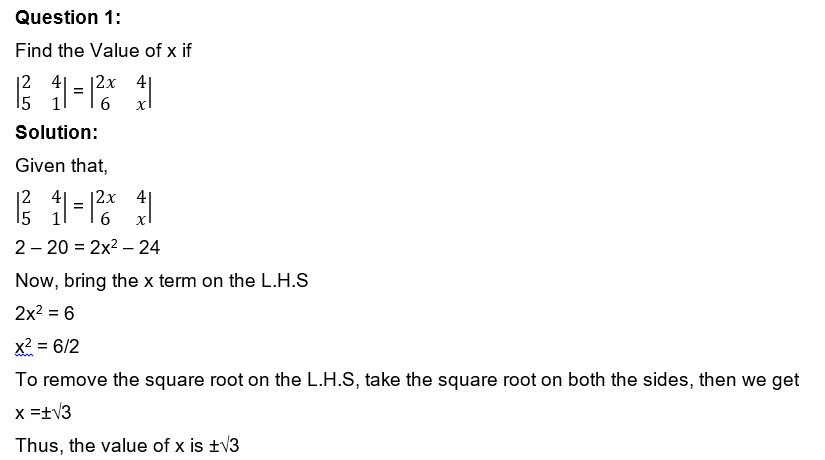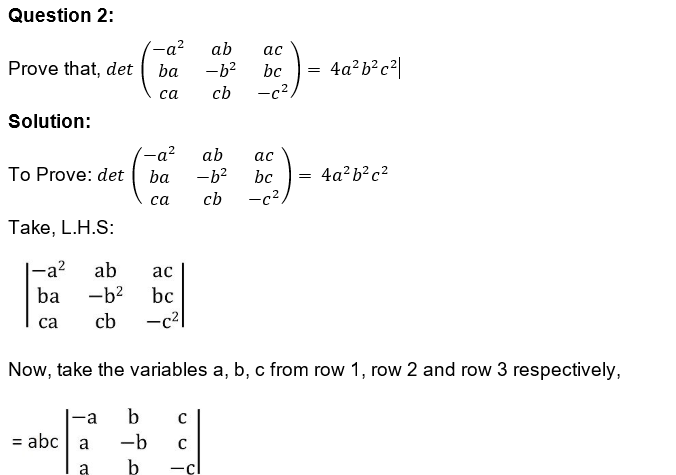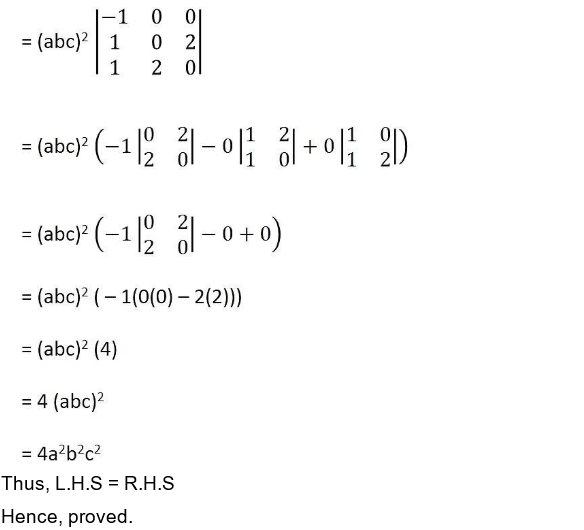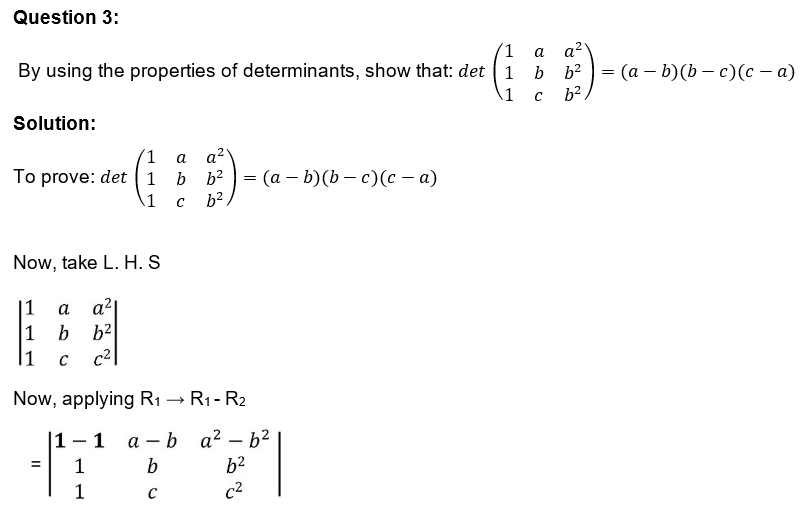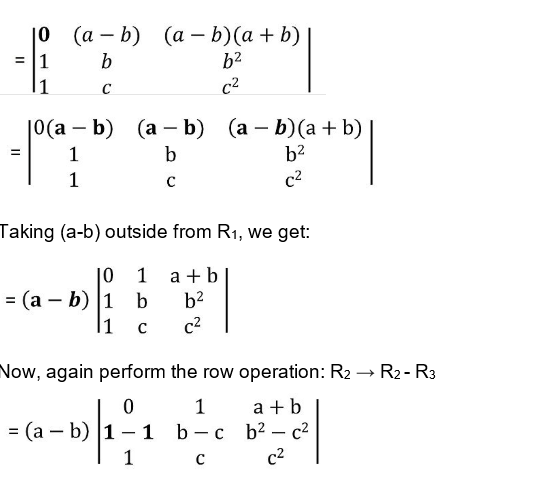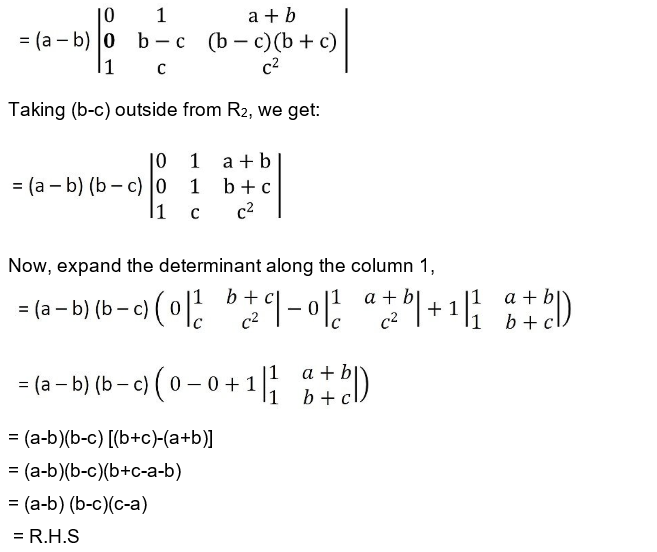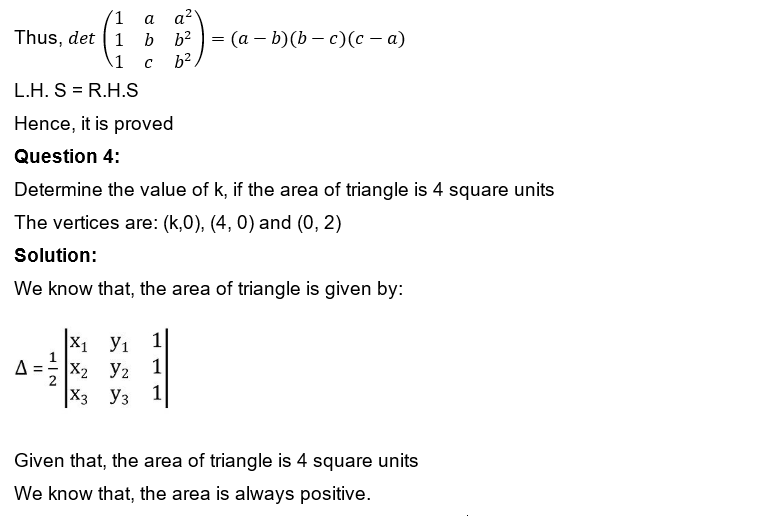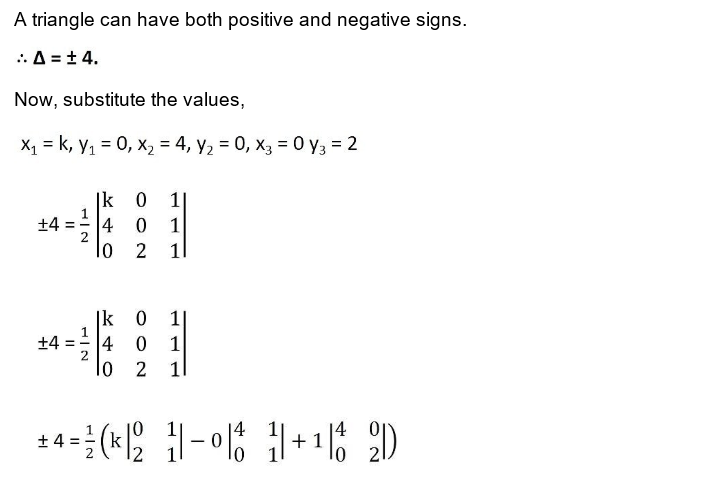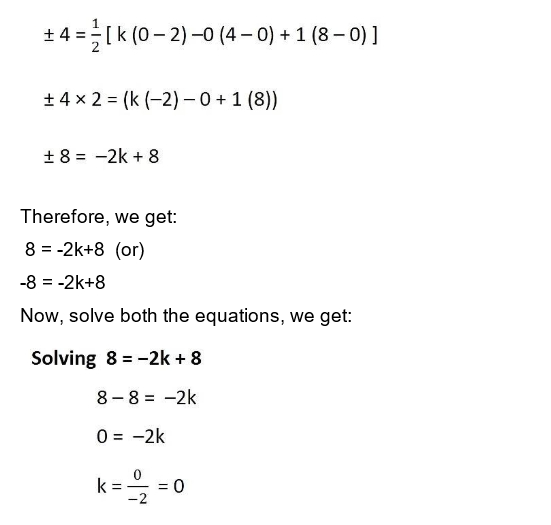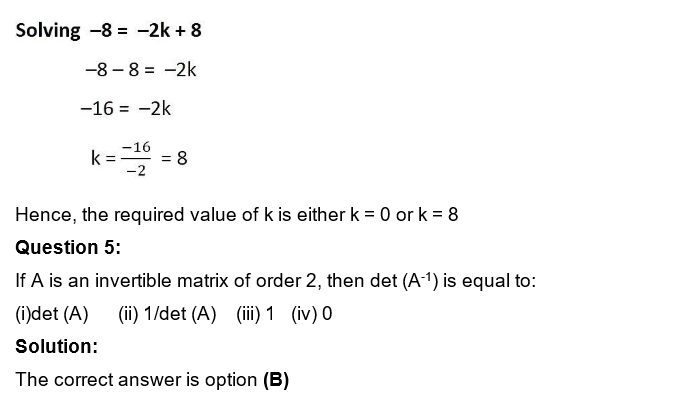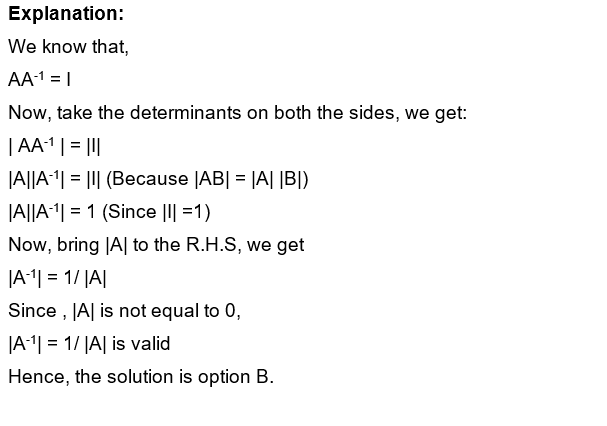### Practice Problem for Class 12 Maths Chapter 4

Solve the practice problems given below:

1. Using the properties of determinants, prove that:
$$\begin{array}{l}\begin{vmatrix} 1 & x &x^{2} \\ x^{2}& 1 & x\\ x& x^{2} &1 \end{vmatrix} = (1-x^{3})^{2}\end{array}$$
2. Find the inverse of each of the matrices if it exists.
$$\begin{array}{l}\begin{bmatrix} 1 &-1 &2 \\ 0&2 &-3 \\ 3& -2 & 4 \end{bmatrix}\end{array}$$
3. Show that the points A(a, b+c), B(b,c+a), C (c, a+b) are collinear.
4. The cost of 4 kg onion, 3 kg wheat and 2 kg rice is Rs 60. The cost of 2 kg onion, 4 kg wheat and 6 kg rice is Rs 90. The cost of 6 kg onion 2 kg wheat and 3 kg rice is Rs 70. Find the cost of each item per kg by matrix method.
5.  Find the equation of the line joining A(1, 3) and B(0, 0) using determinants and find k if D(k, 0) is a point such that area of triangle ABD is 3 sq units.

For more important questions for class 12 Maths, visit BYJU’S – The Learning App and download the app to learn with ease.